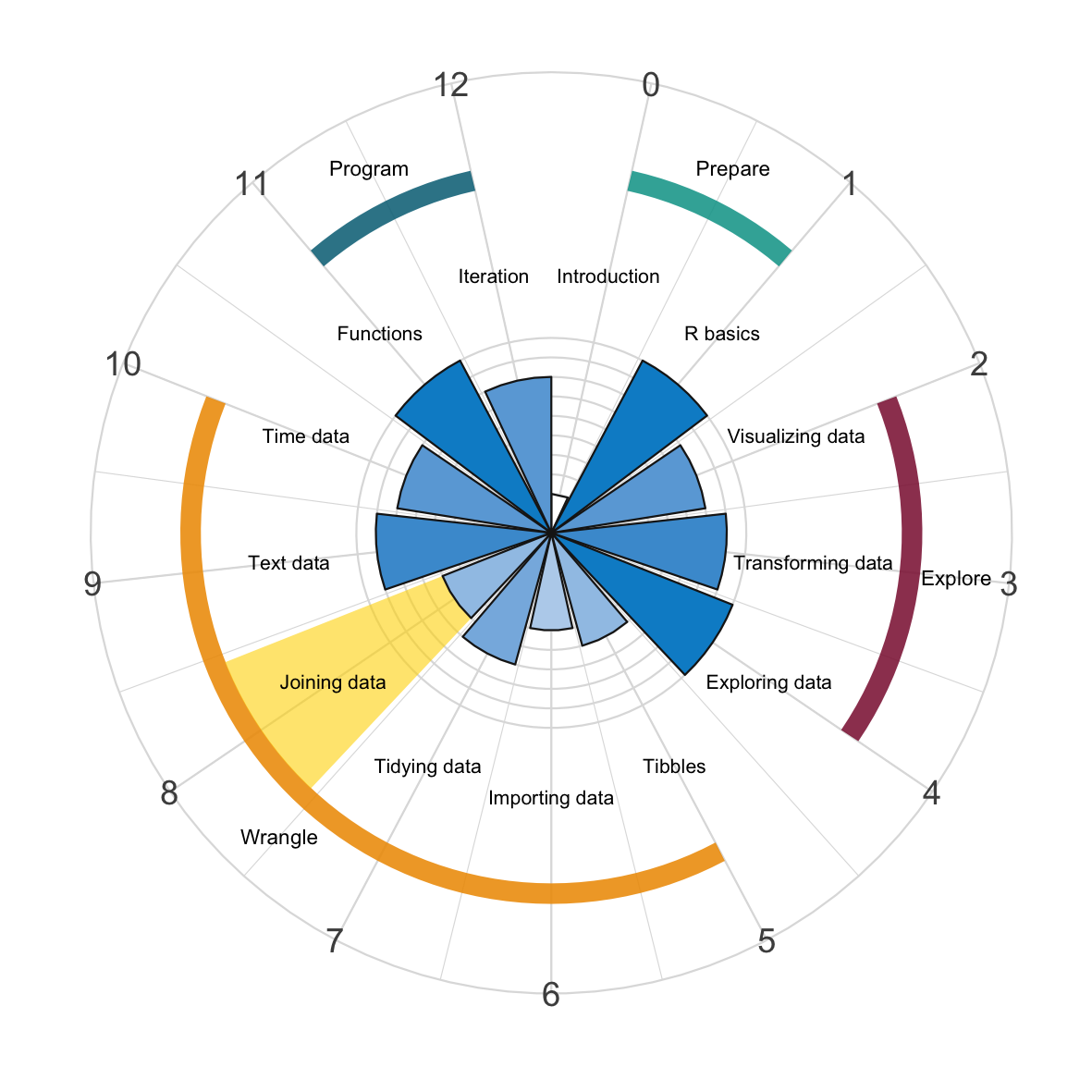# Chapter 8 Joining dataA serious limitation of our data wrangling experiences so far is that they only involved a single table of data. In real settings, this is rarely the case: Any serious data science project is likely to involve multiple tables that need to be combined or linked to answer our questions. Thus, in this chapter, we will start with two tables and learn to join them in various ways. This is still relatively simple, but can easily be generalized to working with multiple tables.Figure 8.1: Joining data with dplyr is a central chapter of the data wrangling part of this book.

This chapter is a central chapter in the Data wrangling part of this book. Whereas the following chapters address particular types of data, this chapter takes up core aspects of Data transformation (see Chapter 3), but adjusts to the reality that we may have imported (see Chapter 6) and tidied (see Chapter 7) multiple tibbles (see Chapter 5) at this point.

The commands that we will use for joining two tables are implemented in the dplyr package , which we already encountered as a core citizen of the tidyverse . In addition to the so-called one-table verbs that we discussed in Chapter 3 on Data transformation, we will now learn additional two-table verbs that allow combining the variables (columns) or cases (rows) of two tables x and y.